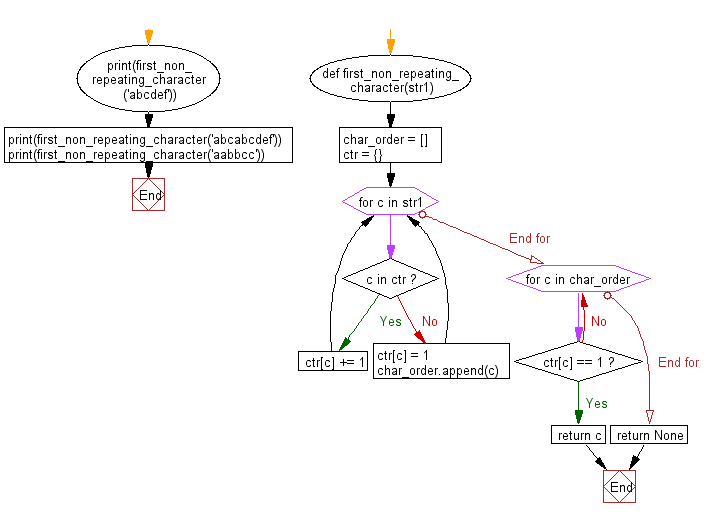﻿ Python: Find the first non-repeating character in given string - w3resource# Python: Find the first non-repeating character in given string

## Python String: Exercise-51 with Solution

Write a Python program to find the first non-repeating character in given string.

Sample Solution:-

Python Code:

``````def first_non_repeating_character(str1):
char_order = []
ctr = {}
for c in str1:
if c in ctr:
ctr[c] += 1
else:
ctr[c] = 1
char_order.append(c)
for c in char_order:
if ctr[c] == 1:
return c
return None

print(first_non_repeating_character('abcdef'))
print(first_non_repeating_character('abcabcdef'))
print(first_non_repeating_character('aabbcc'))
```
```

Sample Output:

```a
d
None
```

Pictorial Presentation:Flowchart:## Visualize Python code execution:

The following tool visualize what the computer is doing step-by-step as it executes the said program:

Python Code Editor:

Have another way to solve this solution? Contribute your code (and comments) through Disqus.

What is the difficulty level of this exercise?

Test your Programming skills with w3resource's quiz.

﻿

## Python: Tips of the Day

What is the difference between Python's list methods append and extend?

append: Appends object at the end.

```x = [1, 2, 3]
x.append([4, 5])
print (x)
```

Output:

```[1, 2, 3, [4, 5]]
```

extend: Extends list by appending elements from the iterable.

```x = [1, 2, 3]
x.extend([4, 5])
print (x)
```

Output:

```[1, 2, 3, 4, 5]
```

Ref: https://bit.ly/2AZ6ZFq